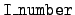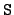Next: Kinematics type 3 Up: The format for `mcf.bin' Previous: Kinematics type 1   Contents

## Kinematics type 2

This kinematics type usually refers to reactions with more than two final particles, and all of the data is in the laboratory system. Energy distributions (= 4 data) and energy depositions (= 10 data) are given for all particles. Uncorrelated angular distributions (= 1 data) and multiplicities (= 7 or 9 data) may also be given. Specifically, a type 2 kinematics directory takes the following form.

Directory length:
The length of this kinematics directory.

For each particle:
A block with the following segments. (The number of particles is given in the reaction directory.)

Identification of secondary particle:
Identify the secondary particle by yo number.

Energy bins:
Location of the= 4 data.

Angular bins:
Location of the= 1 data if it is present in the file. Otherwise, these four numbers are zero.

Energy deposition:
Location of the= 10 data.

Multiplicity:
There are three multiplicity blocks, one for photons (= 9), one for prompt fission neutrons (= 7 and= 0) and one for delayed fission neutrons (= 7 and= 7).

Reserved:
Two slots per particle are reserved for future use.Next: Kinematics type 3 Up: The format for `mcf.bin' Previous: Kinematics type 1   Contents